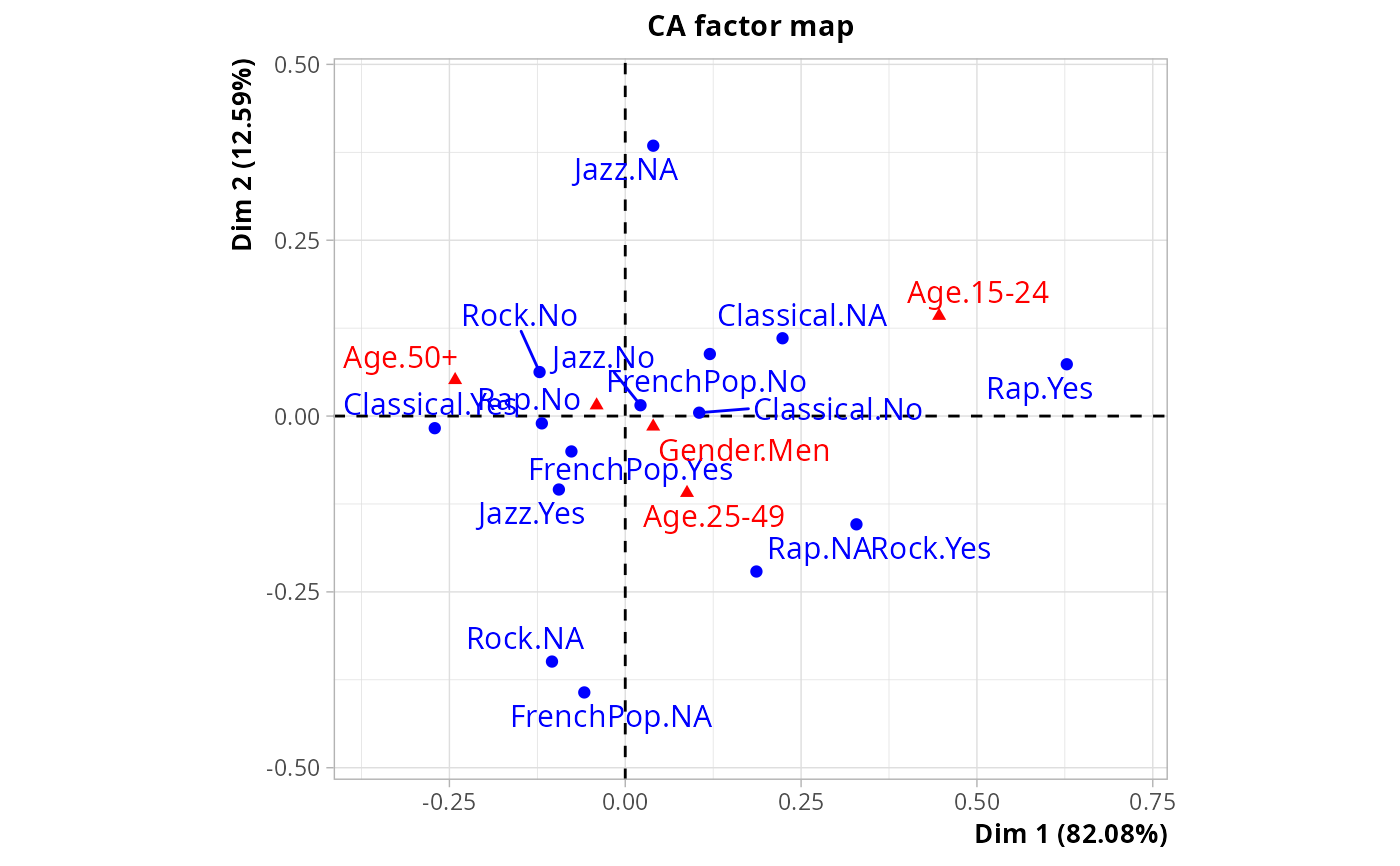Coinertia analysis between two groups of categorical variables

coiMCA(Xa, Xb,
excl.a = NULL, excl.b = NULL,
row.w = NULL, ncp = 5)

## Arguments

Xa

data frame with the first group of categorical variables

Xb

data frame with the second group of categorical variables

excl.a

numeric vector indicating the indexes of the "junk" categories in Xa (default is NULL). See getindexcat or use ijunk interactive function to identify these indexes. It may also be a character vector of junk categories, specified in the form "namevariable.namecategory" (for instance "gender.male").

excl.b

numeric vector indicating the indexes of the "junk" categories in Xb (default is NULL). See excl.a argument.

row.w

numeric vector of row weights. If NULL (default), a vector of 1 for uniform row weights is used.

ncp

number of dimensions kept in the results (by default 5)

## Details

Coinertia analysis aims at capturing the structure common to two groups of variables. With groups of numerical variables, it is equivalent to Tucker's inter-battery analysis. With categorical data, it consists in the following steps : 1. Transformation of Xa and Xb into indicator matrices (i.e. disjunctive tables) Xad and Xbd 2. Computation of the covariance matrix t(Xad).Xbd 3. CA of the matrix

## Value

An object of class CA from FactoMineR package, with an additional item :

RV

the RV coefficient between the two groups of variabels

## Author

Nicolas Robette

coiPCA, rvcoef

## Examples

data(Music)
# music tastes
Xa <- Music[,1:5]
# gender and age
Xb <- Music[,6:7]
# coinertia analysis
res <- coiMCA(Xa, Xb)
plot(res)# RV coefficient
res\$RV
#>  0.06064611